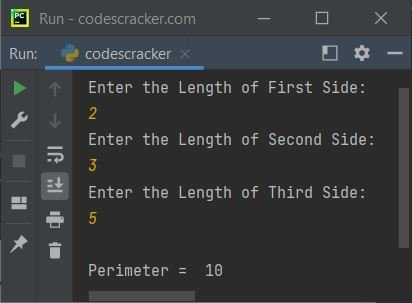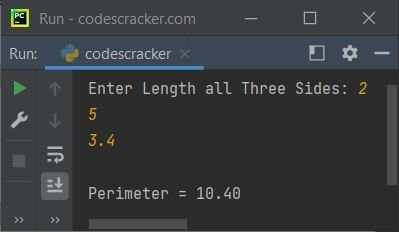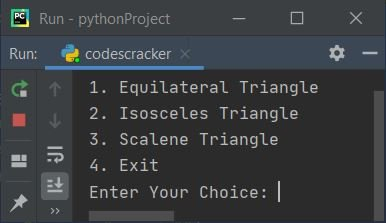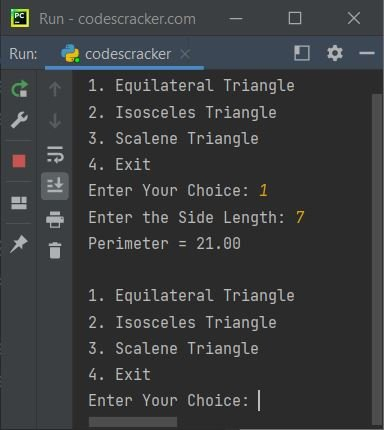# Python Program to Find Perimeter of Triangle

In this article, we've created some programs in Python, to find and print the perimeter value of a triangle based on inputs such as length of all three sides, length of base and height etc. by user at run-time. Here are the list of programs:

• Find Perimeter of Triangle with 3 Sides
• Find Perimeter of All Types of Triangle
• Find Perimeter of Triangle using Function
• Using Class

Before starting these programs, if you're not aware about formula used to find perimeter of triangle, refer to Perimeter of Triangle Formula to get every required thing.

Note - Perimeter of a triangle can be calculated as summing up all its three sides, that is length_of_first_side + length_of_second_side + length_of_third_side.

## Find Perimeter of Triangle with 3 Sides

This Python program asks from user to enter the length of all three sides of triangle, to find the perimeter value of that triangle. The question is, write a Python program to find and print perimeter of triangle. Here is its answer:

```print("Enter the Length of First Side: ")
a = int(input())
print("Enter the Length of Second Side: ")
b = int(input())
print("Enter the Length of Third Side: ")
c = int(input())

p = a+b+c
print("\nPerimeter = ", p)```

Here is its sample run:Above is the initial output. Now enter the length of all three sides one by one say 2 as length of first, 3 as length of second, and 5 as length of third side. Press `ENTER` key to find and print the perimeter value as shown in the snapshot given below:#### Modified Version of Previous Program

In this program, using {:.2f} with format(), we've restricted the value of p to output upto only two decimal places. And the end is used to skip an automatic newline using print().

```print("Enter Length all Three Sides: ", end="")
a = float(input())
b = float(input())
c = float(input())

p = a+b+c
print("\nPerimeter = {:.2f}".format(p))```

Here is its sample run with user input, 2, 5, 3.4 as length of all three sides of triangle:#### Another Modified Version of Previous Program

This program uses list to store length of all three sides of triangle. Rest of the things are similar to previous program. The append() method is used to append element to the list.

```print("Enter Length all Three Sides: ", end="")
TriangleSides = []
p = 0
for i in range(3):
TriangleSides.append(float(input()))
p = p+TriangleSides[i]

print("\nPerimeter = {:.2f}".format(p))```

This program produces exactly same output as of previous program.

### Find Perimeter of all Types of Triangle

Note - Based on the side length of triangle, there are three types of triangles. That are, Scalene, Isosceles, and Equilateral triangle.

```ch = 1
while ch>=1 and ch<=3:
print("1. Equilateral Triangle")
print("2. Isosceles Triangle")
print("3. Scalene Triangle")
print("4. Exit")
ch = int(input())
if ch==1:
print("Enter the Side Length: ", end="")
s = float(input())
p = 3*s
print("Perimeter = {:.2f}\n".format(p))
elif ch==2:
print("Enter Length of any one Side from Two Equal Sides: ", end="")
a = float(input())
print("Enter Length of Third Side: ", end="")
b = float(input())
p = (2*a)+b
print("Perimeter = {:.2f}\n".format(p))
elif ch==3:
print("Enter Length of all Three Sides: ", end="")
a = []
p = 0
for i in range(3):
a.append(float(input()))
p = p + a[i]
print("Perimeter = {:.2f}\n".format(p))
elif ch!=4:
print("Invalid Input!")
print("Try again...\n")
ch = 1```

Here is its initial output:Now enter 1 as choice if you want to find perimeter of an equilateral triangle. Here is its sample run with user choice as 1 and 7 as length of side:This process continues, until you type 4 as choice to exit from the loop.

## Find Perimeter of Triangle using Function

This program is created using user-defined function named perOfTriangle() that returns perimeter value of a triangle based on the length of all three sides entered by user.

```def perOfTriangle(x, y, z):
return x+y+z

print("Enter Length all Three Sides: ", end="")
a = float(input())
b = float(input())
c = float(input())

p = perOfTriangle(a, b, c)
print("\nPerimeter = {:.2f}".format(p))```

## Find Perimeter of Triangle using Class

This is the last program of this article, created using class, an object-oriented feature of Python to do the same job as previous program. Let's have a look at the program:

```class CodesCracker:
def perOfTriangle(self, x, y, z):
return x+y+z

print("Enter Length all Three Sides: ", end="")
a = float(input())
b = float(input())
c = float(input())

ob = CodesCracker()
p = ob.perOfTriangle(a, b, c)
print("\nPerimeter = {:.2f}".format(p))```

An object named ob is created of type CodesCracker class to access its member function through dot (.) operator. Rest of the things are similar to normal function.

Python Online Test

« Previous Program Next Program »